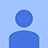# Đề thi Olympic Tiếng Anh lớp 9 vòng 1 năm 2013 - 2014

1 753

## Đề thi Olympic Tiếng Anh lớp 9 vòng 1

Đề thi Olympic Tiếng Anh (IOE) lớp 9 vòng 1 năm học 2013 - 2014 có đáp án kèm theo được VnDoc sưu tầm, chọn lọc giúp các em học sinh ôn tập và củng cố kiến thức, nhằm đạt kết quả cao trong các vòng tiếp theo của cuộc thi Olympic giải Tiếng Anh qua mạng năm học 2013 - 2014. Sau đây mời các em cùng tham khảo.

### Đề thi Olympic Tiếng Anh lớp 9 vòng 1 năm 2013 - 2014

Chọn câu trả lời tốt nhất cho những câu hỏi dưới đây.

1. The value of x is even, and the value of y is odd. Which expression will produce an even number?

A. x + 2y B. 2x + y C. x2 + y2 D. (x + y)2 E. x + y

2. Similar trapezoids are shown. What is the value of n?

A. 10 B. 12 C. 15 D. 19 E. 22

3. What does the expression 0.5 × 6 × 4.5 + 10.52 + 62 equal?

A. 46.5 B. 135.75 C. 159.75 D. 285.75 E. 5514. The areas in square miles of the Great Lakes are shown in the bar graph. Based on this graph, which set of numbers is the closest to the total area of the five Great Lakes?

A. between 70,000 and 80,000 square miles
B. between 80,000 and 90,000 square miles
C. between 90,000 and 100,000 square miles
D. between 100,000 and 110,000 square miles
E. between 1,000 and 11,000 square miles

5. Mr. Radke took a survey and found the mean, mode, median, and range of fishing-boat lengths in his community. Which measure represents the fishing-boat length most often found?

A. mean B. median C. mode D. range E. None of them6. Points R and T are the midpoints of the sides of triangle QSU, as shown in the diagram. What is the perimeter of ΔRST?

A. 26 B. 40 C. 54 D. 80 E. 99

7. Keisha is 6 feet tall. How tall, to the nearest meter, is Keisha?

A. 2 B. 3 C. 9 D. 20 E. 358. George keeps track of the shots on goal that his hockey team takes each game. The table shows the data. Based on the trend in the table, which is the best prediction of shots on goal for the next game?

A. 3 B. 7 C. 12 D. 22 E. 30

9. The number of hamburgers sold at a local restaurant varies inversely with the price that is charged. The number, n, of hamburgers sold at a price, p, in dollars can be found using the formula n = 687.5/p. Approximately how many hamburgers did the restaurant sell if the price of the hamburgers was \$3?

A. 278 B. 229 C. 225 D. 215 E. 245

10. Which property does the equation 24(70 + 5) = 24(70) + 24(5) illustrate?

A. associative B. commutative

C. distributive D. identity

E. None of them

## Đáp án đề thi Olympic Tiếng Anh lớp 9 vòng 1

Câu 1: C Câu 2: D Câu 3: A Câu 4: C Câu 5: D

Câu 6: C Câu 7: B Câu 8: C Câu 9: B Câu 10: D

Đánh giá bài viết
1 753
0 Bình luận
Sắp xếp theo